# GregorioTeX snippets Tutorial

Documentation for the gabc notation which we use in this tutorial can be found here. A one-page summary is also on-line (PDF).

The purpose of this tutorial is to demonstrate how to represent a Gregorian chant score in a gabc snippet directly in your TeX file. This method is only recommended for short, simple scores taking up no more than a line or two.

If you plan on following along with the tutorial, you'll need to choose your method for using Gregorio and set your system up first.

We'll start with an empty staff (with a clef) and build up from there. Along the way, I'll make use of some useful tricks which help speed up and ensure the accuracy of the transcription. I'll also make a few common mistakes in order to illustrate some potential pitfalls.

Here is a score from the Antiphonale Monasticum.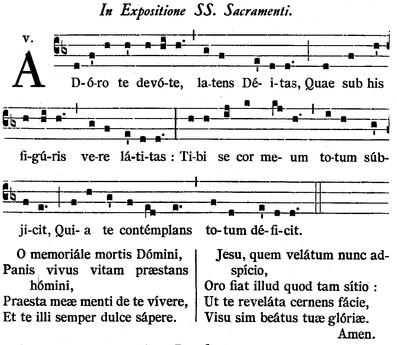Since this score is so simple, we're going to make use of the \gabcsnippet command here. This will allow us to keep all our work in a single file. However, this choice does come with some limitations (which we will discuss later) and so is not suitable for all scores. For a tutorial on how to make use of a separate file to represent the score, see our other tutorial.

To start this project off, let's create our file and look at its initial output:

\documentclass[12pt, a5paper]{article}

\usepackage{fullpage}

\usepackage{fontspec}
\usepackage{libertine}

\usepackage[autocompile]{gregoriotex}

\begin{document}

\gabcsnippet{(cb3)}

\end{document}


Save this file as adoro-te-main.tex (in order to differentiate between the different steps, we'll add the step number to this file name (as "adoro-te-01-main.tex" above), but if you're following along you can ignore this) and then compile it using LuaLaTeX.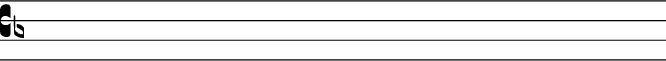For the next few steps, we're going to be adding material exclusively to the \gabcsnippet line, so I'm only going to show that line when noting the changes.

Since the text and the notes are interlaced in gabc, it is difficult to spell check one's transcription after both are on the page. To avoid this, we'll start by adding the text by itself and then come back and add in the notes later. So our first step looks something like this:

\gabcsnippet{(cb3) Adoro te devote, latens Deitas,
Quae sub his figuris vere latitas:
Tibi se cor meum totum subjicit,
Quia te contemplans totum deficit.}


Since we've left out the notes, we can run this block of text through a Latin language spell-checker at this point to make sure we've gotten our transcription right. Depending on your system setup, this may require copying the text into another program and then copying back any corrections.

Once we've double checked the text to make sure it's correct, it's time to syllabify it by adding () after each syllable. For something this short, this is relatively easy to do manually, but for a longer text you might want to explore using the syllabifier in the hyphen-la project.

Regardless of what method you choose for syllabifying the text, you should end up with something like this:

\gabcsnippet{(cb3) Ad()or()o() te() de()vo()te,() la()tens() De()i()tas,()
Quae() sub() his() fi()gu()ris() ve()re() la()ti()tas:()
Ti()bi() se() cor() me()um() to()tum() sub()ji()cit,()
Qui()a() te() con()tem()plans() to()tum() de()fi()cit.()}


Compiling this document gives us: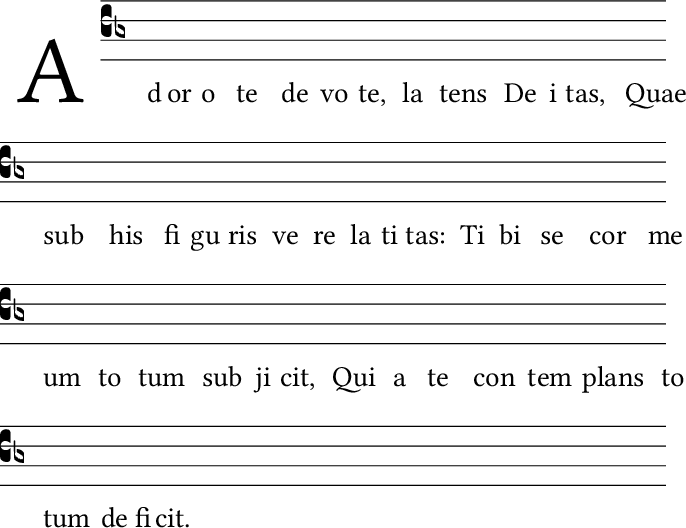You'll note that the words at the end of each line hang out into the margin (past the staff lines). That, however, is not something to worry about at this point. It is merely the result of all our syllables having no notes in them yet and will fix itself when we add the notes.

Speaking of which, let's do that now:

\gabcsnippet{(cb3) Ad(d)or(f’)o(h) te(h’) de(h)vo(hi)te,(h.) la(g)tens(f) De(e’)i(d)tas,(d.)
Quae(d) sub(f) his(h’) fi(h)gu(hi)ris(h.) ve(g)re(f) la(e’)ti(d)tas:(d.)
Ti(h)bi(h) se(ij) cor(k) me(kj)um(h.) to(i)tum(h) sub(g’)ji(f)cit,(e.)
Qui(f)a(h) te(g’) con(f)tem(ed)plans(d.) to(f)tum(d) de(e’)fi(d)cit.(d.)}


If I compile this file, however, I get a whole bunch of “unrecognized character” errors (which are non-fatal) and none of the vertical episema show up in my score. This is becuase I had “Smart Quote” replacement active in my editor and so when I typed ' (the straight quote) what I actually got was ’ (a curly right quote). This is fixable with a simple find and replace, but it would also be a good idea to turn off “Smart Quote” replacement when editing gabc.

Having fixed that error, my file now looks like this:

\gabcsnippet{(cb3) Ad(d)or(f')o(h) te(h') de(h)vo(hi)te,(h.) la(g)tens(f) De(e')i(d)tas,(d.)
Quae(d) sub(f) his(h') fi(h)gu(hi)ris(h.) ve(g)re(f) la(e')ti(d)tas:(d.)
Ti(h)bi(h) se(ij) cor(k) me(kj)um(h.) to(i)tum(h) sub(g')ji(f)cit,(e.)
Qui(f)a(h) te(g') con(f)tem(ed)plans(d.) to(f)tum(d) de(e')fi(d)cit.(d.)}


And it compiles to: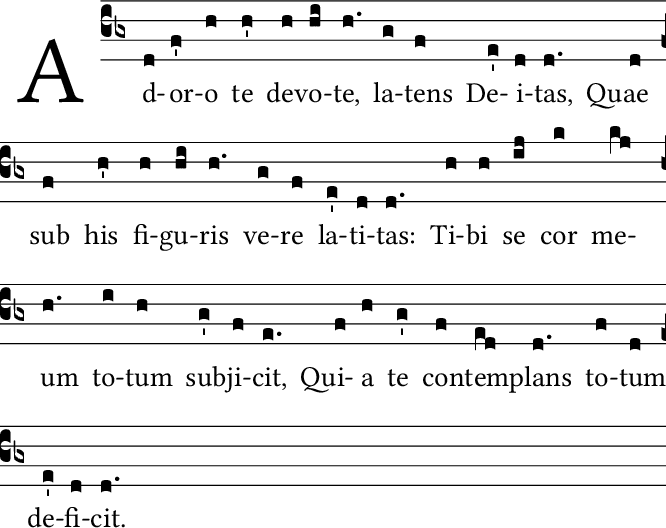As promised, the text no longer hangs out into the margin.

To complete this score, we simply need to add in the bar lines:

\gabcsnippet{(cb3) Ad(d)or(f')o(h) te(h') de(h)vo(hi)te,(h.) (,) la(g)tens(f) De(e')i(d)tas,(d.) (;)
Quae(d) sub(f) his(h') fi(h)gu(hi)ris(h.) (,) ve(g)re(f) la(e')ti(d)tas:(d.) (:)
Ti(h)bi(h) se(ij) cor(k) me(kj)um(h.) (,) to(i)tum(h) sub(g')ji(f)cit,(e.) (;)
Qui(f)a(h) te(g') con(f)tem(ed)plans(d.) (,) to(f)tum(d) de(e')fi(d)cit.(d.) (::)}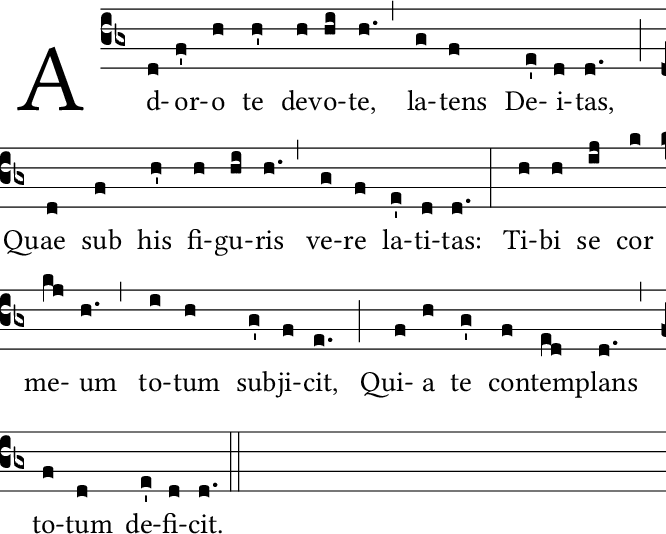Looking over the apparently complete score, I just realized that I forgot the accents in some of the words. To add these in, I need to go back and replace the appropriate unaccented vowels with their accented counterparts. This should be doable either in unicode (i.e. entering é directly), or through TeX code (i.e. entering {\'e}), as is illustrated below:

\gabcsnippet{(cb3) Ad(d)ór(f')o(h) te(h') de(h)vó(hi)te,(h.) (,) la(g)tens(f) Dé(e')i(d)tas,(d.) (;)
Quae(d) sub(f) his(h') fi(h)gú(hi)ris(h.) (,) ve(g)re(f) lá(e')ti(d)tas:(d.) (:)
Ti(h)bi(h) se(ij) cor(k) me(kj)um(h.) (,) to(i)tum(h) s{\'u}b(g')ji(f)cit,(e.) (;)
Qui(f)a(h) te(g') con(f)t{\'e}m(ed)plans(d.) (,) to(f)tum(d) d{\'e}(e')fi(d)cit.(d.) (::)}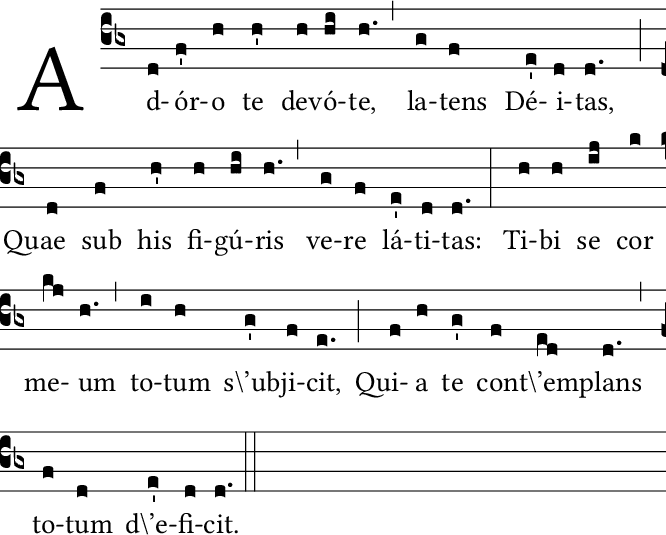However, as our result clearly illustrates, only the first method works exactly as expected. This is because the contents of the \gabcsnippet command must follow the rules of gabc for the entering of TeX code by setting it off in <v></v> tags:

\gabcsnippet{(cb3) Ad(d)ór(f')o(h) te(h') de(h)vó(hi)te,(h.) (,) la(g)tens(f) Dé(e')i(d)tas,(d.) (;)
Quae(d) sub(f) his(h') fi(h)gú(hi)ris(h.) (,) ve(g)re(f) lá(e')ti(d)tas:(d.) (:)
Ti(h)bi(h) se(ij) cor(k) me(kj)um(h.) (,) to(i)tum(h) s<v>{\'u}</v>b(g')ji(f)cit,(e.) (;)
Qui(f)a(h) te(g') con(f)t<v>{\'e}</v>m(ed)plans(d.) (,) to(f)tum(d) d<v>{\'e}</v>(e')fi(d)cit.(d.) (::)}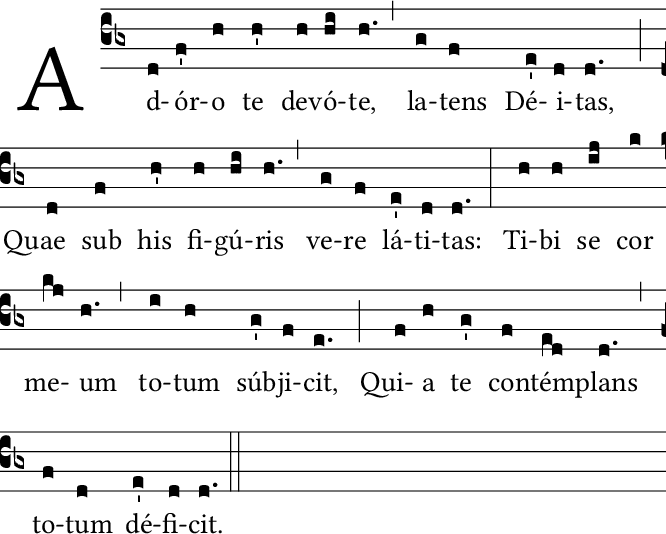If the need to explicitly mark TeX code as such within a .tex file seems counter-intuitive, you must remember that the rules for gabc syntax were developed under the original assumption that it would be in its own separate file, not embedded directly in a .tex file (via the \gabcsnippet command). This is one of the limitations of the \gabcsnippet command that I mentioned earlier. Even though we're in a .tex file, we still have to follow the rules for gabc, even if they don't make as much sense in this context.

To complete our score, we need to add in the mode number. If we were creating a .gabc file, when we could enter this information as part of the file header and GregorioTeX would pick this information up and print it properply formatted for us. However, one of the other limitations of the \gabcsnippet command is that there is no place for the header, so we must do all of this ourselves manually with the \greannoation command:

\documentclass[12pt, a5paper]{article}

\usepackage{fullpage}

\usepackage{fontspec}
\usepackage{libertine}

\usepackage[autocompile]{gregoriotex}

\begin{document}

\greannotation{\textsc{v}}

\gabcsnippet{(cb3) Ad(d)ór(f')o(h) te(h') de(h)vó(hi)te,(h.) (,) la(g)tens(f) Dé(e')i(d)tas,(d.) (;)
Quae(d) sub(f) his(h') fi(h)gú(hi)ris(h.) (,) ve(g)re(f) lá(e')ti(d)tas:(d.) (:)
Ti(h)bi(h) se(ij) cor(k) me(kj)um(h.) (,) to(i)tum(h) s<v>{\'u}</v>b(g')ji(f)cit,(e.) (;)
Qui(f)a(h) te(g') con(f)t<v>{\'e}</v>m(ed)plans(d.) (,) to(f)tum(d) d<v>{\'e}</v>(e')fi(d)cit.(d.) (::)}

\end{document}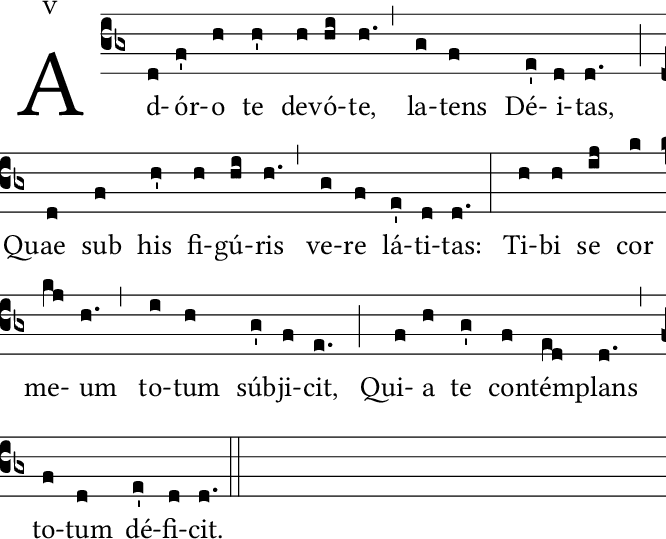With that our score is finished (the addition of the title and the text of the two subsequent verses are left as an exercise for the reader). Before leaving the tutorial, however, we should mention the other two major limitations of the \gabcsnippet command:

• because the header is not available, you cannot define custom macros, but must enter the whole macro each time you want to use it.
• you cannot have any mismatched braces in your gabc code. Any mismatched brace will cause TeX to have difficutly telling where the \gabcsnippet command ends. As a result, you must be extremely careful when using a forced syllable center, using dynamicly sized braces, or simple polyphony as all of these will introduce braces into your gabc syntax. While all should work if done correctly, the more braces you introduce into your code, the more potential problem points there are.
• Because of these limitations, our advice is that you only use the \gabcsnippet command for simple chants which don't take up more than a few lines.